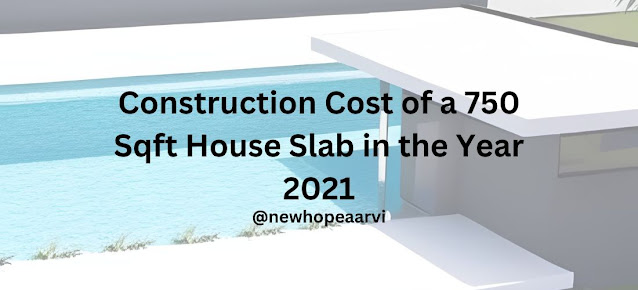## Posts

Showing posts with the label Rate Analysis

### Construction Cost of a 750 Sqft House Slab in the Year 2021How to find out the Construction Cost of a 750 Sqft House Slab in the Year 2021? Here we go for the steps we need to take care of for finding the Construction Cost of a 750 Sqft House Slab in the Year 2021 Volume Calculations Table-1 Material Calculations Qty of Cement Required Please Note: Always Use M20 (1:1.5:3) Grade of Concrete for Slab Total Ratio = 1+1.5+3 = 5.5 Now, converting the quantity from cum to kg, we need the density of the cement in the unit of the kg/cum, and the density of the cement is 1440kg/cum As we know that 50 kgs of the cement make 1 bag, hence, 3567.942 kgs in bags will be 71.359 bags Qty of Sand Required To convert the cum of sands in cft, we have to multiply the quantity by 35.314, hence, the sand required in cft will be 131.248 cft Qty of Aggregates Required Converting the cum to cft, we should multiply the quantity by 35.314, hence, the aggregates required in cft will be 262.496 cft Qty of Reinf Steel Required Considering the qty of steel, 0.5% to 1.00%